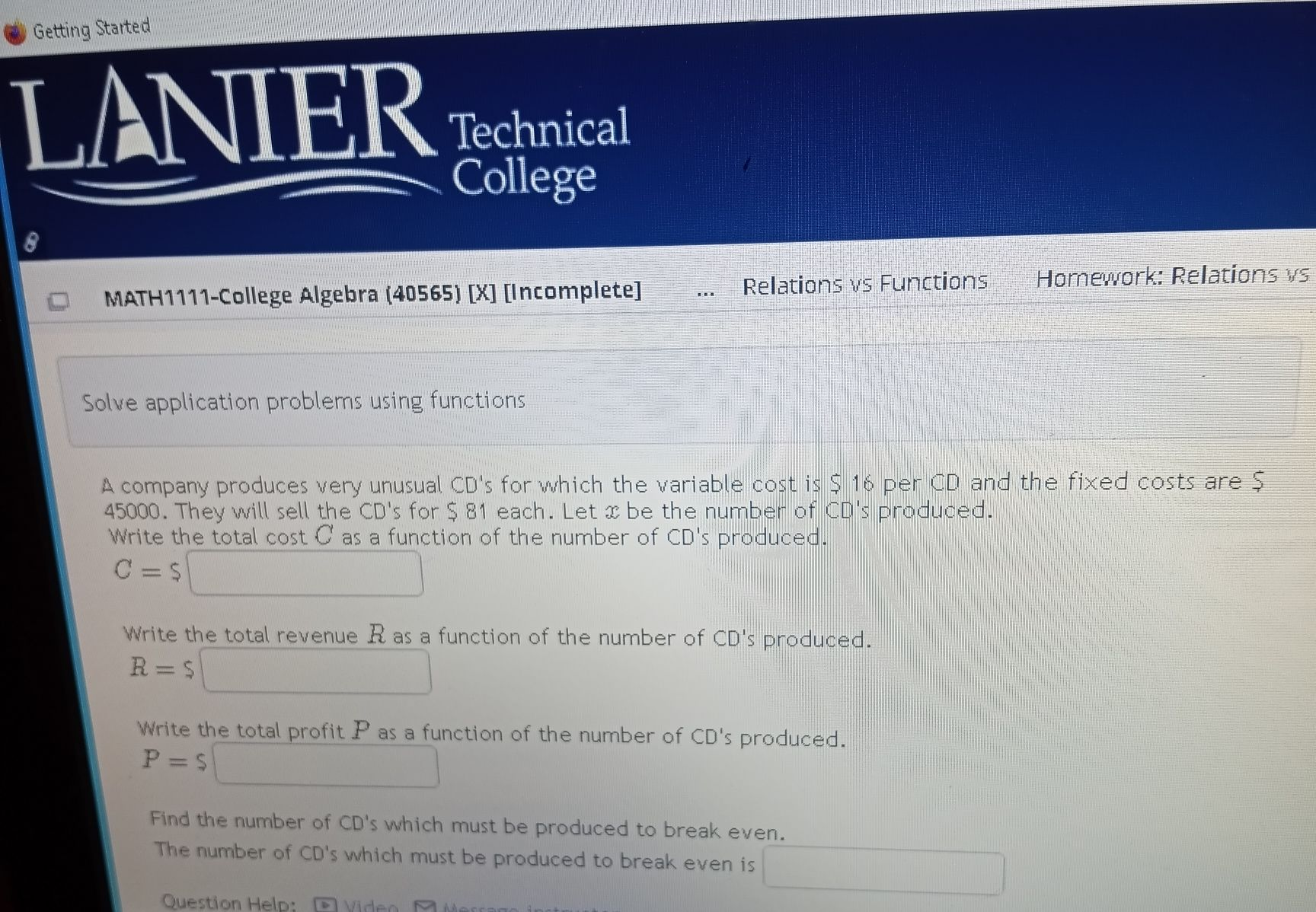### Still have math questions?

Algebra
QuestionA company produces very unusual CD's for which the variable cost is $$\ 16$$ per CD and the fixed costs are  $$\ 45000$$ . They will sell the CD's for $$\ 81$$ each. Let $$x$$ be the number of CD's produced. Write the total cost $$C$$ as a function of the number of CD's produced.

$$C = \$$

Write the total revenue $$R$$ as a function of the number of CD's produced.

$$R = \$$

Write the total profit $$P$$ as a function of the number of CD's produced.

$$P = \$$

Find the number of CD's which must be produced to break even.

The number of CD's which must be produced to break even is

$$C= 45000+ 16x$$

$$R= 81x$$

$$P= 81x- ( 45000+ 16x)$$

$$= 65x- 45000$$

Number of CD's to be sold to break even is $$692$$

Solution
View full explanation on CameraMath App.